# NR Interference Modeling with Toroidal Wrap-Around

This example models a 19-cell cluster with toroidal wrap-around as described in ITU-R M.2101-0. The wrap-around provides uniform interference at the cluster edge. All the cells in the cluster operate in the same frequency band with the serving gNB at the center of the cell. You can enable or disable the wrap-around to observe that with wrap-around, the performance metrics of an edge cell becomes similar to the center cell.

### Introduction

The example considers these operations within the gNB and user equipment (UE).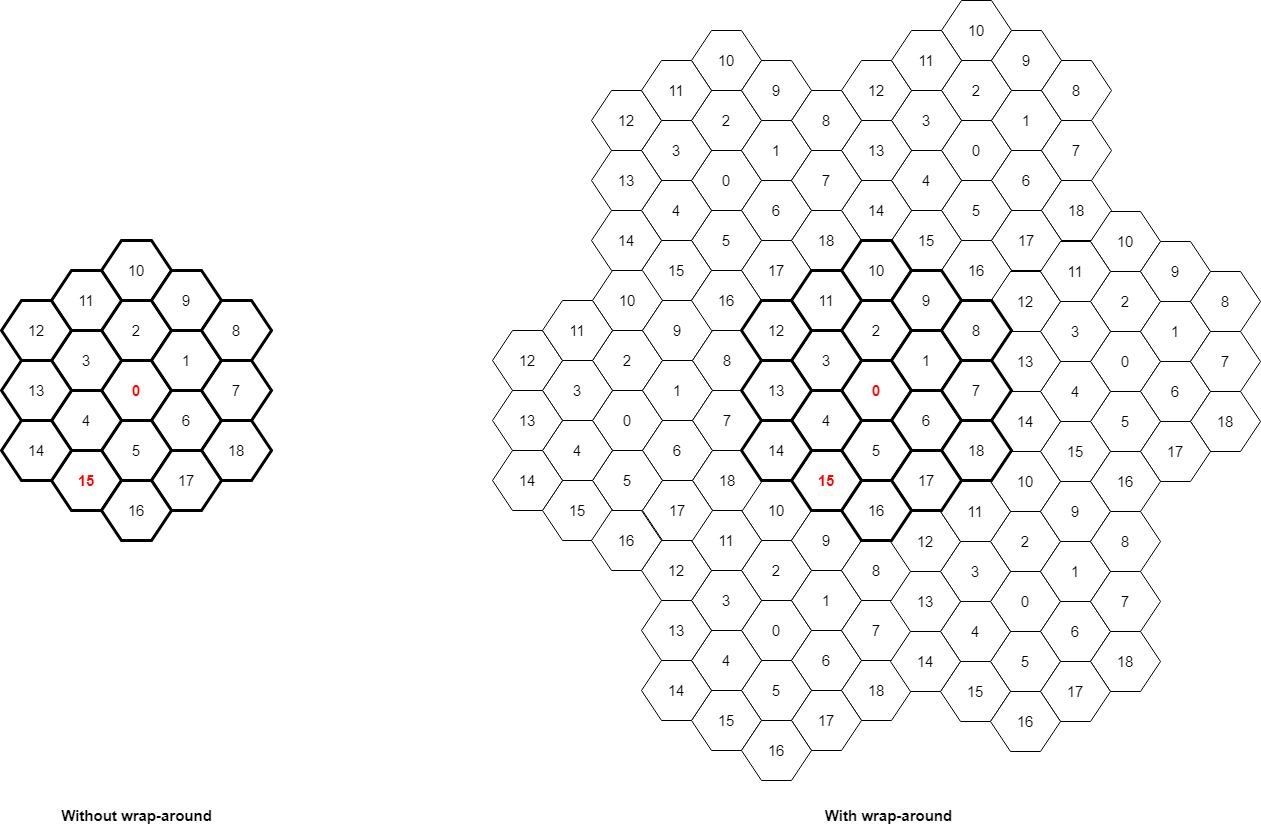This example models:

• Co-channel intercell interference with wrap-around modeling for removing edge effects

• PUSCH demodulation reference signal (DM-RS) and PDSCH DM-RS

• DL channel quality measurement by UEs based on the CSI-RS received from gNB.

• Single-input single-output (SISO) configuration with omnidirectional antennas.

• Free space path loss (FSPL), and additive white Gaussian noise (AWGN).

The example assumes that control packets such as UL assignment, DL assignment, buffer status report (BSR), PDSCH feedback, and channel quality indicator (CQI) report, are sent out of band, that is, without the need of resources for transmission and assured error-free reception.

### Toroidal Wrap-around Modeling

To simulate the behavior of a cellular network without introducing edge effects, this example models an infinite cellular network by using toroidal wrap-around. This figure shows the network region of 19 cells. In the absence of wrap-around, cell 0 of the central cluster, shown in red, is uniformly surrounded and experiences interference from all sides. An edge cell like cell-15 experiences comparatively lesser interference. The wrap-around repeats the original cluster six times to uniformly surround the central cluster.

The entire network region relevant for simulations is a cluster of 19 cells (shown in bold).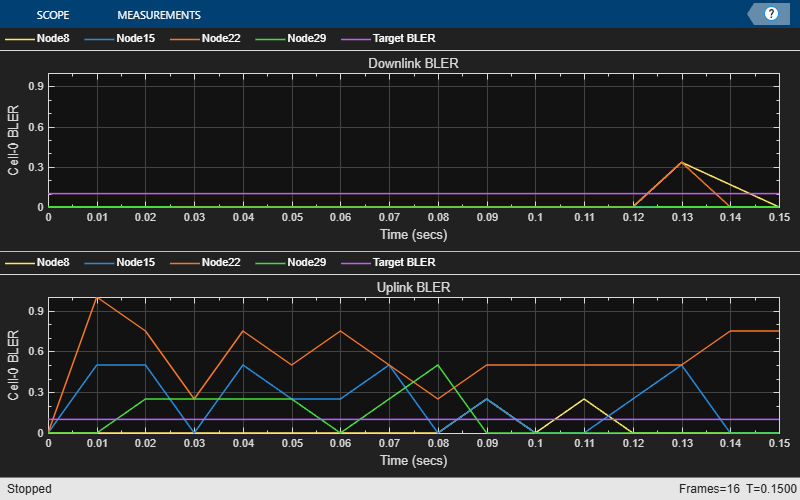In the wrap-around model, the signal or interference from any UE to a cell is treated as if that UE is in the original cell cluster and the gNB in any of the seven clusters as specified in ITU-R M.2101-0. The distances used to compute the path loss from a transmitter node at $\left(a,b\right)$ to a receiver node at $\left(x,y\right)$ is the minimum of these seven distances.

• Distance between $\left(x,y\right)$ and $\left(a,b\right)$

• Distance between $\left(x,y\right)$ and $\left(a-\sqrt{3}D,b-4D\right)$, where $D$ is the distance between two adjacent gNBs (inter-site distance)

• Distance between $\left(x,y\right)$ and $\left(a+\sqrt{3}D,b+4D\right)$

• Distance between $\left(x,y\right)$ and $\left(a-\frac{\sqrt{3}}{2}D,b+\frac{7}{2}D\right)$

• Distance between $\left(x,y\right)$ and $\left(a+\frac{3\sqrt{3}}{2}D,b-\frac{7}{2}D\right)$

• Distance between $\left(x,y\right)$ and $\left(a-\frac{5\sqrt{3}}{2}D,b-\frac{1}{2}D\right)$

• Distance between $\left(x,y\right)$ and $\left(a-\frac{5\sqrt{3}}{2}D,b-\frac{1}{2}D\right)$

To calculate the modified distances between nodes due to the wrap-around, the function uses the hNRNodeDistanceCalculator helper class. If you disable wrap-around, then the distance between nodes is the normal Euclidean distance.

#### NR Protocol Stack

A node (gNB or UE) consists of applications generating traffic, a radio link control (RLC) layer, a medium access control (MAC) layer, and a physical layer (PHY). The hNRGNB and hNRUE helper classes create gNB and UE nodes, respectively, containing RLC, MAC, and PHY layers. For more details on each layer, refer to the 'NR Protocol Stack' section in the NR Cell Performance Evaluation with Physical Layer Integration example.

### Scenario Configuration

Configure simulation parameters in the `simParameters` structure.

```rng('default'); % Reset the random number generator simParameters = []; % Clear the simParameters variable simParameters.NumFramesSim = 5; % Simulation time, in number of 10 ms frames simParameters.EnableWrapAround = true; % Enable wrap-around modeling simParameters.SchedulingType = 0; % Slot-based scheduling```

Specify the number of UEs in each cell, assuming that UEs have sequential radio network temporary identifiers (RNTIs) from `1` to `simParameters.NumUEsCell`. If you change the number of UEs, you must ensure that these simulation parameters are arrays of length equal to the value of `simParameters.NumUEsCell`: `simParameters.UEDistance`, `ulPacketPeriodicity`, `ulPacketSizes`, `dlPacketPeriodicity`, `dlPacketSizes`.

```simParameters.NumUEsCell = 2; % Number of UEs in each cell simParameters.UEDistance = [150; 250]; % Distance of UE from gNB in meters simParameters.InterSiteDistance = 1732; % Distance between adjacent gNBs in meters```

Set the channel bandwidth to 5 MHz and the subcarrier spacing (SCS) to 15 kHz as defined in section 5.3.2 of 3GPP TS 38.104.

```simParameters.NumRBs = 25; simParameters.SCS = 15; % kHz simParameters.DLCarrierFreq = 2.635e9; % Hz simParameters.ULCarrierFreq = 2.515e9; % Hz```

Assume that the UL and DL carriers have the same channel bandwidth, and specify the transmit power and antenna gain.

```simParameters.DLBandwidth = 5e6; % Hz simParameters.ULBandwidth = 5e6; % Hz simParameters.UETxPower = 23; % Tx power for all the UEs in dBm simParameters.GNBTxPower = 32; % Tx power for gNBs in dBm simParameters.GNBRxGain = 8; % Receiver antenna gain at gNB in dBi```

Specify the signal-to-interference-plus-noise ratio (SINR) to CQI mapping table for a block error rate (BLER) of 0.1.

```simParameters.SINR90pc = [-5.46 -0.46 4.54 9.05 11.54 14.04 15.54 18.04 ... 20.04 22.43 24.93 25.43 27.43 30.43 33.43];```

Specify the scheduling strategy and the maximum limit on the RBs allotted for PUSCH and PDSCH. The transmission limit applies only to new PUSCH and PDSCH assignments, and not to the retransmissions.

```simParameters.SchedulerStrategy = 'PF'; % Supported scheduling strategies: 'PF', 'RR', and 'BestCQI' simParameters.RBAllocationLimitUL = 20; % For PUSCH simParameters.RBAllocationLimitDL = 20; % For PDSCH ```

#### Logging and Visualization Configuration

Specify the ID of cell of interest as an integer between 1 and 18, inclusive. The example shows visualizations and metrics for this cell and the central cell, cell-0.

```simParameters.CellOfInterest = 15; % Set a value from 1 to 18. Set the value to 0 to visualize cell-0 only. validateattributes(simParameters.CellOfInterest, {'numeric'}, {'integer', 'scalar', '>=', 0, '<=', 18}, 'simParameters.CellOfInterest', 'CellOfInterest')```

The `CQIVisualization` and `RBVisualization` parameters control the display of the CQI visualization of RBs and the RB assignment visualization. To enable the RB visualization plot, set the `RBVisualization` field to `true`.

```simParameters.CQIVisualization = true; simParameters.RBVisualization = false;```

Update the output metrics plots periodically, specifying `NumMetricsSteps` updates within the simulation.

`simParameters.NumMetricsSteps = 10;`

Write the logs to MAT-files. The example uses these logs for post-simulation analysis and visualization.

```simParameters.ParametersLogFile = 'simParameters'; % For logging the simulation parameters simParameters.SimulationLogFile = 'simulationLogs'; % For logging the simulation logs```

#### Application traffic configuration

Set the periodic UL and DL application traffic pattern for UEs.

```ulPacketPeriodicity = [10; 10]; % Periodicity, in ms, at which the UEs generate UL packets ulPacketSize = [2e4; 2e4]; % Size of generated UL packets, in bytes dlPacketPeriodicity = [10; 10]; % Periodicity, in ms, at which gNB generates DL packets dlPacketSize = [2e4; 2e4]; % Size of generated DL packets, in bytes```

### Derived Parameters

Compute the derived parameters based on the primary configuration parameters specified in the previous section, and set some example-specific constants.

```simParameters.DuplexMode = 0; % Frequency-division duplexing (FDD) simParameters.NumClusters = 1; simParameters.NumSitesPerCluster = 19; % Number of gNBs per cluster```

Set the UE and gNB positions.

```simParameters.GNBPositions = hMacrocellTopology(simParameters); simParameters.UEPositions = cell(simParameters.NumSitesPerCluster, 1); for idx = 1:simParameters.NumSitesPerCluster theta = rand(simParameters.NumUEsCell, 1)*(2*pi); % Set the UE position with respect to the gNB location simParameters.UEPositions{idx} = simParameters.GNBPositions(idx, :) + simParameters.UEDistance.*[cos(theta) sin(theta) zeros(simParameters.NumUEsCell, 1)]; end simParameters.NCellIDList = 0:simParameters.NumSitesPerCluster-1; % List of physical cell IDs```

Specify the CSI-RS resource configuration, assuming that all UEs measure channel quality on the same CSI-RS resource.

```simParameters.CSIRSRowNumber = 2; % Possible row numbers for single transmit antenna case are 1 and 2 simParameters.SubbandSize = 8; % Size of sub-band for CQI reporting in terms of number of RBs```

Calculate the slot duration for the selected SCS and the number of slots in a 10 ms frame.

```slotDuration = 1/(simParameters.SCS/15); % In ms numSlotsFrame = 10/slotDuration; % Number of slots in a 10 ms frame numSlotsSim = simParameters.NumFramesSim*numSlotsFrame; % Number of slots in the simulation```

Set the interval at which the example updates metrics visualization, number of slots. Because this example uses a time granularity of one slot, the `MetricsStepSize` field must be an integer.

```simParameters.MetricsStepSize = ceil(numSlotsSim / simParameters.NumMetricsSteps); if mod(numSlotsSim, simParameters.NumMetricsSteps) ~= 0 % Update the NumMetricsSteps parameter if it does not exactly divide NumSlotsSim simParameters.NumMetricsSteps = floor(numSlotsSim / simParameters.MetricsStepSize); end```

Specify one logical channel in each UE, and set the logical channel configuration for all nodes (UEs and gNBs) in the example.

```numLogicalChannels = 1; simParameters.LCHConfig.LCID = 4; % Logical channel ID (logical channel ID of data radio bearers starts from 4)```

Specify the RLC entity direction as `0` to specify DL only, `1` to specify UL only, or `2` to specify both UL and DL.

`simParameters.RLCConfig.EntityDir = 2;`

Create RLC channel configuration structure.

```rlcChannelConfigStruct.LCGID = 1; % Mapping between logical channel and logical channel group ID rlcChannelConfigStruct.Priority = 1; % Priority of each logical channel rlcChannelConfigStruct.PBR = 8; % Prioritized bitrate (PBR), in kilobytes per second, of each logical channel rlcChannelConfigStruct.BSD = 10; % Bucket size duration (BSD), in ms, of each logical channel rlcChannelConfigStruct.EntityType = simParameters.RLCConfig.EntityDir; rlcChannelConfigStruct.LogicalChannelID = simParameters.LCHConfig.LCID;```

Set the maximum RLC service data unit (SDU) length, in bytes, as specified in 3GPP TS 38.323, and the total number of nodes in the simulation.

```simParameters.maxRLCSDULength = 9000; simParameters.MaxReceivers = simParameters.NumSitesPerCluster*(simParameters.NumUEsCell + 1); if ~isfield(simParameters, 'SchedulingType') || simParameters.SchedulingType == 0 % No scheduling type or slot-based scheduling rbAssignmentPlotPeriodicity = numSlotsFrame; % Update RB assignment visualization every frame (10 ms) tickGranularity = 14; simParameters.PUSCHMappingType = 'A'; simParameters.PDSCHMappingType = 'A'; else % Symbol-based scheduling rbAssignmentPlotPeriodicity = 1; % Update RB assignment visualization every slot tickGranularity = 1; simParameters.PUSCHMappingType = 'B'; simParameters.PDSCHMappingType = 'B'; end```

### Multicell Setup

Set up the 19-cell topology with each cell consisting of one gNB and one UE. For each cell, create the gNB and UE objects, initialize the channel quality information for UEs, and set up the logical channel at gNB and UEs. The hNRGNB and hNRUE helper classes create gNB and UE nodes respectively, containing the RLC, MAC, and PHY layers.

```gNB = cell(simParameters.NumSitesPerCluster, 1); UEs = cell(simParameters.NumSitesPerCluster, simParameters.NumUEsCell);```

Create DL and UL packet distribution objects, and initialize a wrap-around distance calculator object.

```dlPacketDistributionObj = hNRPacketDistribution(simParameters, 0); % 0 for DL ulPacketDistributionObj = hNRPacketDistribution(simParameters, 1); % 1 for UL distCalc = hNRNodeDistanceCalculator(simParameters); for siteIdx = 1:simParameters.NumSitesPerCluster simParameters.NCellID = simParameters.NCellIDList(siteIdx); % Cell ID simParameters.Position = simParameters.GNBPositions(siteIdx, :); simParameters.NumUEs = simParameters.NumUEsCell; % Number of UEs in a cell % Create scheduler switch(simParameters.SchedulerStrategy) case 'RR' % Round robin scheduler scheduler = hNRSchedulerRoundRobin(simParameters); case 'PF' % Proportional fair scheduler scheduler = hNRSchedulerProportionalFair(simParameters); case 'BestCQI' % Best CQI scheduler scheduler = hNRSchedulerBestCQI(simParameters); end % Create gNB gNB{siteIdx} = hNRGNB(simParameters); addScheduler(gNB{siteIdx}, scheduler); % Add scheduler to gNB gNB{siteIdx}.PhyEntity = hNRGNBPhy(simParameters); % Create PHY layer instance configurePhy(gNB{siteIdx}, simParameters); % Configure PHY layer % Register distance calculator for wrap-around distance computations gNB{siteIdx}.DistanceCalculatorFcn = @distCalc.getDistance; setPhyInterface(gNB{siteIdx}); % Set up the interface to PHY layer for ueIdx = 1:simParameters.NumUEsCell simParameters.Position = simParameters.UEPositions{siteIdx}(ueIdx, :); % Position of UE in (x,y,z) coordinates UEs{siteIdx, ueIdx} = hNRUE(simParameters, ueIdx); UEs{siteIdx, ueIdx}.PhyEntity = hNRUEPhy(simParameters, ueIdx); % Create PHY layer instance configurePhy(UEs{siteIdx, ueIdx}, simParameters); % Configure PHY layer % Register distance calculator for wrap-around distance computations UEs{siteIdx, ueIdx}.DistanceCalculatorFcn = @distCalc.getDistance; setPhyInterface(UEs{siteIdx, ueIdx}); % Set up the interface to PHY % Set up logical channel at gNB for the UE configureLogicalChannel(gNB{siteIdx}, ueIdx, rlcChannelConfigStruct); % Set up logical channel at UE configureLogicalChannel(UEs{siteIdx, ueIdx}, ueIdx, rlcChannelConfigStruct); % Add data traffic pattern generators to gNB and UE nodes % Calculate the data rate (in kbps) of On-Off traffic pattern using % packet size (in bytes) and packet interval (in ms) ulDataRate = ceil(1000/ulPacketPeriodicity(ueIdx)) * ulPacketSize(ueIdx) * 8e-3; % Limit the size of the generated application packet to the maximum RLC % SDU size. The maximum supported RLC SDU size is 9000 bytes if ulPacketSize(ueIdx) > simParameters.maxRLCSDULength ulPacketSize(ueIdx) = simParameters.maxRLCSDULength; end % Create an object for On-Off network traffic pattern and add it to the % specified UE. This object generates the uplink data traffic on the UE ulApp = networkTrafficOnOff('PacketSize', ulPacketSize(ueIdx), 'GeneratePacket', true, ... 'OnTime', simParameters.NumFramesSim/100, 'OffTime', 0, 'DataRate', ulDataRate); UEs{siteIdx, ueIdx}.addApplication(ueIdx, simParameters.LCHConfig.LCID, ulApp); dlDataRate = ceil(1000/dlPacketPeriodicity(ueIdx)) * dlPacketSize(ueIdx) * 8e-3; if dlPacketSize(ueIdx) > simParameters.maxRLCSDULength dlPacketSize(ueIdx) = simParameters.maxRLCSDULength; end % Create an object for On-Off network traffic pattern for the specified % UE and add it to the gNB. This object generates the downlink data % traffic on the gNB for the UE dlApp = networkTrafficOnOff('PacketSize', dlPacketSize(ueIdx), 'GeneratePacket', true, ... 'OnTime', simParameters.NumFramesSim/100, 'OffTime', 0, 'DataRate', dlDataRate); gNB{siteIdx}.addApplication(ueIdx, simParameters.LCHConfig.LCID, dlApp); end % Setup the UL and DL packet distribution mechanism hNRSetUpPacketDistribution(simParameters, gNB{siteIdx}, UEs(siteIdx, :), dlPacketDistributionObj, ulPacketDistributionObj); end```

Set up logging and visualization, specifying the central cell (cell 0) and the cell of interest.

```cellsOfInterest = unique([0; simParameters.CellOfInterest]); numCellsOfInterest = length(cellsOfInterest); % Number of cells that the example logs and visualizes```

Visualize the network topology

`hTopologyVisualizer(simParameters); `Log and visualize PHY and MAC metrics in cell arrays.

```simSchedulingLogger = cell(numCellsOfInterest, 1); % Cell array for PHY metrics logging and visualization simPhyLogger = cell(numCellsOfInterest, 1); visualizer = cell(numCellsOfInterest, 1); for siteIdx = 1:numCellsOfInterest simParameters.NCellID = cellsOfInterest(siteIdx); simParameters.CellOfInterest = simParameters.NCellID; % Create an object for MAC scheduling information visualization and logging simSchedulingLogger{siteIdx} = hNRSchedulingLogger(simParameters); % Create an object for PHY layer metrics logging simPhyLogger{siteIdx} = hNRPhyLogger(simParameters); % Create visualization object for MAC and PHY metrics visualizer{siteIdx} = hNRMetricsVisualizer(simParameters, 'MACLogger', simSchedulingLogger{siteIdx}, 'PhyLogger', simPhyLogger{siteIdx}); end```

### Processing Loop

Simulation is run slot by slot. For each cell, in each slot, these operations are executed:

• Run the MAC and PHY layers of gNB

• Run the MAC and PHY layers of UEs

• Log and visualize metrics for each layer

• Advance the timer for the nodes and send a trigger to application and RLC layers every millisecond. The application layer and RLC layer execute their scheduled operations based on a 1 ms timer trigger.

```slotNum = 0; numSymbolsSim = numSlotsSim * 14; % Simulation time in units of symbol duration % Run processing loop for symbolNum = 1 : tickGranularity : numSymbolsSim if mod(symbolNum - 1, 14) == 0 slotNum = slotNum + 1; end % Because all the cells operate on the same SCS, slot durations do not vary for siteIdx = 1:simParameters.NumSitesPerCluster % For each site run(gNB{siteIdx}); % Run MAC and PHY layers of UEs for ueIdx = 1:simParameters.NumUEsCell run(UEs{siteIdx, ueIdx}); end cellIdx = find((siteIdx-1) == cellsOfInterest, 1); if ~isempty(cellIdx) % MAC logging logCellSchedulingStats(simSchedulingLogger{cellIdx}, symbolNum, gNB{siteIdx}, UEs(siteIdx, :)); % PHY logging logCellPhyStats(simPhyLogger{cellIdx}, symbolNum, gNB{siteIdx}, UEs(siteIdx, :)); end end for siteIdx = 1:simParameters.NumSitesPerCluster % Advance timer ticks for gNB and UEs by the number of symbols per slot advanceTimer(gNB{siteIdx}, tickGranularity); for ueIdx = 1:simParameters.NumUEsCell advanceTimer(UEs{siteIdx, ueIdx}, tickGranularity); end end for idx = 1:numCellsOfInterest % Visualization % Check slot boundary if symbolNum > 1 && ((simParameters.SchedulingType == 1 && mod(symbolNum, 14) == 0) || (simParameters.SchedulingType == 0 && mod(symbolNum-1, 14) == 0)) % RB assignment visualization (if enabled) if simParameters.RBVisualization if mod(slotNum, rbAssignmentPlotPeriodicity) == 0 % Plot at slot boundary, if the update periodicity is reached plotRBGrids(simSchedulingLogger{idx}); end end % CQI grid visualization (if enabled) if simParameters.CQIVisualization if mod(slotNum, numSlotsFrame) == 0 % Plot at frame boundary plotCQIRBGrids(simSchedulingLogger{idx}); end end % If the update periodicity is reached, plot scheduler metrics and PHY metrics visualization % at slot boundary if mod(slotNum, simParameters.MetricsStepSize) == 0 plotMetrics(visualizer{idx}, slotNum); end end end end```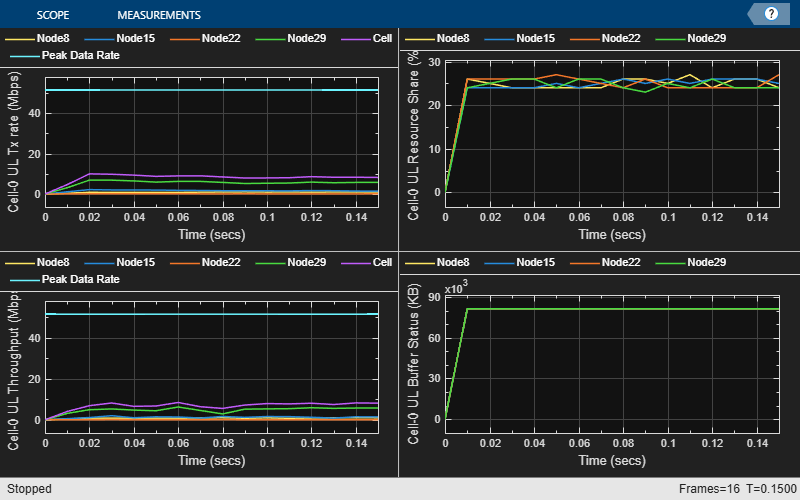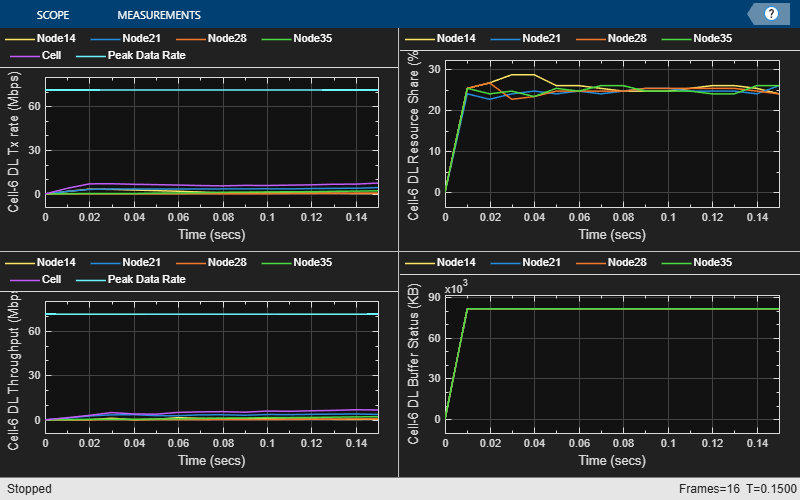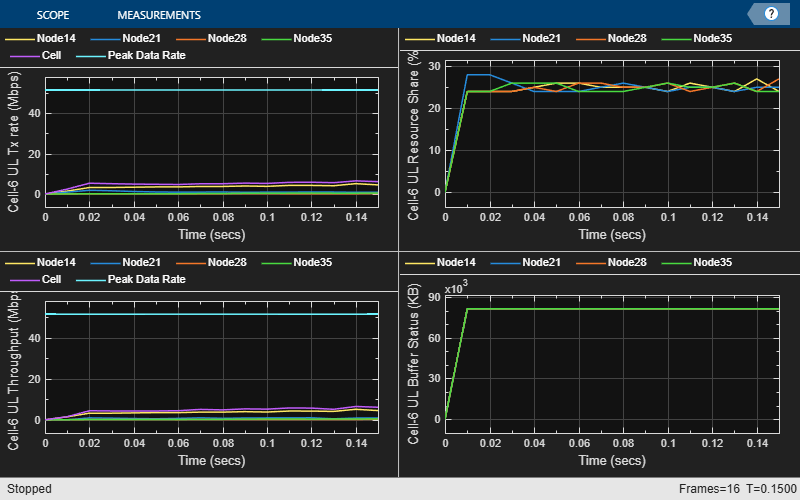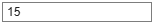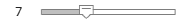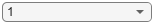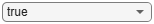```for idx = 1:numCellsOfInterest [dlStats, ulStats] = getPerformanceIndicators(simSchedulingLogger{idx}); [logInfo.BLERLogs, logInfo.AvgBLERLogs] = getBLERLogs(simPhyLogger{idx}); % Block Error rate logs fprintf('\n\nMetrics for cell %d :\n\n', cellsOfInterest(idx)); fprintf('Peak UL throughput: %0.2f Mbps. Achieved average UL Throughput: %0.2f Mbps', ulStats(1, 1), ulStats(2, 1)); fprintf('\nPeak DL throughput: %0.2f Mbps. Achieved average DL Throughput: %0.2f Mbps', dlStats(1, 1), dlStats(2, 1)); fprintf('\nAchieved average UL Goodput: %0.2f Mbps. Achieved average DL Goodput: %0.2f Mbps', ulStats(5, 1), dlStats(5, 1)); fprintf('\nPeak UL spectral efficiency: %0.2f bits/s/Hz. Achieved average UL spectral efficiency: %0.2f bits/s/Hz ', ulStats(3, 1), ulStats(4, 1)); fprintf('\nPeak DL spectral efficiency: %0.2f bits/s/Hz. Achieved average DL spectral efficiency: %0.2f bits/s/Hz', dlStats(3, 1), dlStats(4, 1)); disp(['Block error rate for each UE in the uplink direction: [' num2str(round(logInfo.AvgBLERLogs(:, 2)', 2)) ']']); disp(['Block error rate for each UE in the downlink direction: [' num2str(round(logInfo.AvgBLERLogs(:, 1)', 2)) ']']); end```
```Metrics for cell 0 : ```
```Peak UL throughput: 31.11 Mbps. Achieved average UL Throughput: 2.48 Mbps ```
```Peak DL throughput: 31.11 Mbps. Achieved average DL Throughput: 3.19 Mbps ```
```Achieved average UL Goodput: 2.48 Mbps. Achieved average DL Goodput: 3.10 Mbps ```
```Peak UL spectral efficiency: 6.22 bits/s/Hz. Achieved average UL spectral efficiency: 0.50 bits/s/Hz ```
```Peak DL spectral efficiency: 6.22 bits/s/Hz. Achieved average DL spectral efficiency: 0.64 bits/s/Hz ```
```Block error rate for each UE in the uplink direction: [0 0] ```
```Block error rate for each UE in the downlink direction: [0.04 0.04] ```
```Metrics for cell 15 : ```
```Peak UL throughput: 31.11 Mbps. Achieved average UL Throughput: 2.69 Mbps ```
```Peak DL throughput: 31.11 Mbps. Achieved average DL Throughput: 3.33 Mbps ```
```Achieved average UL Goodput: 2.68 Mbps. Achieved average DL Goodput: 3.24 Mbps ```
```Peak UL spectral efficiency: 6.22 bits/s/Hz. Achieved average UL spectral efficiency: 0.54 bits/s/Hz ```
```Peak DL spectral efficiency: 6.22 bits/s/Hz. Achieved average DL spectral efficiency: 0.67 bits/s/Hz ```
```Block error rate for each UE in the uplink direction: [0 0.05] ```
```Block error rate for each UE in the downlink direction: [0.04 0.06] ```

### Simulation Visualization

For the center cell (cell 0) and the cell of interest, the run time visualizations show:

The simulation results show that the center cell and edge cell have similar performance metrics with wrap around. Below are the simulation results with no wrap-around. You can observe significant performance difference between center cell and edge cell.### Simulation Logs

The parameters used for simulation and the simulation logs are saved in MAT-files for post simulation analysis and visualization. The simulation parameters are saved in a MAT-file with the file name as the value of configuration parameter `simParameters.ParametersLogFile`. For more details, see the NR Intercell Interference Modeling example.

```% Get the logs if(simParameters.DuplexMode == 0) % FDD logInfo = struct('NCellID', [], 'DLTimeStepLogs', [], 'ULTimeStepLogs', [], 'SchedulingAssignmentLogs', [], 'BLERLogs', [], 'AvgBLERLogs', []); else logInfo = struct('TimeStepLogs', [], 'SchedulingAssignmentLogs', [], 'BLERLogs', [], 'AvgBLERLogs', []); end simulationLogs = cell(simParameters.NumSitesPerCluster, 1); for siteIdx = 1:numCellsOfInterest logInfo.NCellID = cellsOfInterest(siteIdx); if(simParameters.DuplexMode == 0) % FDD [logInfo.DLTimeStepLogs, logInfo.ULTimeStepLogs] = getSchedulingLogs(simSchedulingLogger{siteIdx}); else % TDD logInfo.TimeStepLogs = getSchedulingLogs(simSchedulingLogger{siteIdx}); end logInfo.SchedulingAssignmentLogs = getGrantLogs(simSchedulingLogger{siteIdx}); % Scheduling assignments log [logInfo.BLERLogs, logInfo.AvgBLERLogs] = getBLERLogs(simPhyLogger{siteIdx}); % Block error rate logs simulationLogs{siteIdx, 1} = logInfo; end save(simParameters.ParametersLogFile, 'simParameters'); % Save simulation parameters in a MAT-file save(simParameters.SimulationLogFile, 'simulationLogs'); % Save simulation logs in a MAT-file```

 3GPP TS 38.104. “NR; Base Station (BS) radio transmission and reception.” 3rd Generation Partnership Project; Technical Specification Group Radio Access Network.

 3GPP TS 38.214. “NR; Physical layer procedures for data.” 3rd Generation Partnership Project; Technical Specification Group Radio Access Network.

 3GPP TS 38.321. “NR; Medium Access Control (MAC) protocol specification.” 3rd Generation Partnership Project; Technical Specification Group Radio Access Network.

 3GPP TS 38.322. “NR; Radio Link Control (RLC) protocol specification.” 3rd Generation Partnership Project; Technical Specification Group Radio Access Network.

 3GPP TS 38.323. “NR; Packet Data Convergence Protocol (PDCP) specification.” 3rd Generation Partnership Project; Technical Specification Group Radio Access Network.

 3GPP TS 38.331. “NR; Radio Resource Control (RRC) protocol specification.” 3rd Generation Partnership Project; Technical Specification Group Radio Access Network.

 3GPP TR 37.910. “Study on self evaluation towards IMT-2020 submission.” 3rd Generation Partnership Project; Technical Specification Group Radio Access Network.